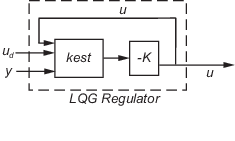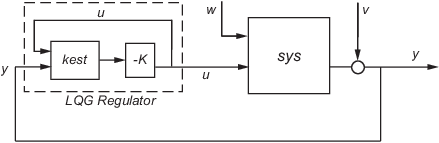Main Content

# lqgreg

Form linear-quadratic-Gaussian (LQG) regulator

## Syntax

```rlqg = lqgreg(kest,k) rlqg = lqgreg(kest,k,controls) ```

## Description

`rlqg = lqgreg(kest,k)` returns the LQG regulator `rlqg` (a state-space model) given the Kalman estimator `kest` and the state-feedback gain matrix `k`. The same function handles both continuous- and discrete-time cases. Use consistent tools to design `kest` and `k`:

• Continuous regulator for continuous plant: use `lqr` or `lqry` and `kalman`

• Discrete regulator for discrete plant: use `dlqr` or `lqry` and `kalman`

• Discrete regulator for continuous plant: use `lqrd` and `kalmd`

In discrete time, `lqgreg` produces the regulator

• $u\left[n\right]=-K\stackrel{^}{x}\left[n|n\right]$ when `kest` is the "current" Kalman estimator

• $u\left[n\right]=-K\stackrel{^}{x}\left[n|n-1\right]$ when `kest` is the "delayed" Kalman estimator

For more information on Kalman estimators, see the `kalman` reference page.

`rlqg = lqgreg(kest,k,controls)` handles estimators that have access to additional deterministic known plant inputs ud. The index vector `controls` then specifies which estimator inputs are the controls u, and the resulting LQG regulator `rlqg` has ud and y as inputs (see the next figure).

Note

Always use positive feedback to connect the LQG regulator to the plant.## Examples

See the example LQG Regulation: Rolling Mill Case Study.

## Algorithms

`lqgreg ` forms the linear-quadratic-Gaussian (LQG) regulator by connecting the Kalman estimator designed with `kalman` and the optimal state-feedback gain designed with `lqr`, `dlqr`, or `lqry`. The LQG regulator minimizes some quadratic cost function that trades off regulation performance and control effort. This regulator is dynamic and relies on noisy output measurements to generate the regulating commands.

In continuous time, the LQG regulator generates the commands

`$u=-K\stackrel{^}{x}$`

where $\stackrel{^}{x}$ is the Kalman state estimate. The regulator state-space equations are

`$\begin{array}{l}\stackrel{˙}{\stackrel{^}{x}}=\left[A-LC-\left(B-LD\right)K\right]\stackrel{^}{x}+Ly\\ u=-K\stackrel{^}{x}\end{array}$`

where y is the vector of plant output measurements (see `kalman` for background and notation). The following diagram shows this dynamic regulator in relation to the plant.In discrete time, you can form the LQG regulator using either the delayed state estimate $\stackrel{^}{x}\left[n|n-1\right]$ of x[n], based on measurements up to y[n–1], or the current state estimate $\stackrel{^}{x}\left[n|n\right]$, based on all available measurements including y[n]. While the regulator

`$u\left[n\right]=-K\stackrel{^}{x}\left[n|n-1\right]$`

is always well-defined, the current regulator

`$u\left[n\right]=-K\stackrel{^}{x}\left[n|n\right]$`

is causal only when I-KMD is invertible (see `kalman` for the notation). In addition, practical implementations of the current regulator should allow for the processing time required to compute u[n] after the measurements y[n] become available (this amounts to a time delay in the feedback loop).

For a discrete-time plant with equations:

`$\begin{array}{c}x\left[n+1\right]=Ax\left[n\right]+Bu\left[n\right]+Gw\left[n\right]\\ y\left[n\right]=Cx\left[n\right]+Du\left[n\right]+Hw\left[n\right]+v\left[n\right]\text{ }\left\{\text{Measurements}\right\}\end{array}$`

connecting the "current" Kalman estimator to the LQR gain is optimal only when $E\left(w\left[n\right]v{\left[n\right]}^{\prime }\right)=0$ and y[n] does not depend on w[n] (H = 0). If these conditions are not satisfied, compute the optimal LQG controller using `lqg`.

## See Also

Introduced before R2006a

## Support

#### Learn how to automatically tune PID controller gains

Download code examples